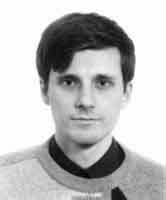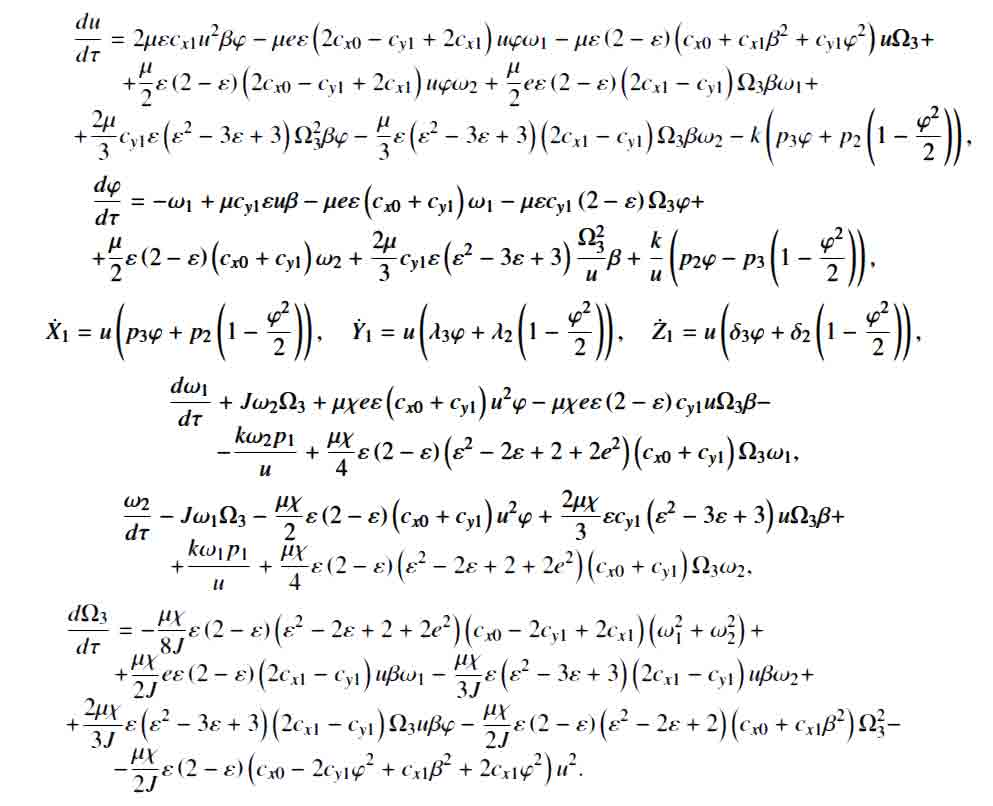# Boomerangs – the aerodynamics ofIt has been said that a fully developed mathematical formula is one of the shortest possible ways to describe a physical phenomenon. Some phenomena, however, are so complex that their mathematical description can be dauntingly large. Take for example the formula to describe the aerial motion of a boomerang.It has been single handedly developed by Alexander S. Kuleshov, at the Department of Mechanics and Mathematics, of  Московского государственного университета.

“Formulation of aerodynamic forces and moments is based on the strip theory, which proved to be quite efficient in problems of dynamics of a rigid body moving in the medium. Equations of motion of the boomerang are derived and investigated numerically.“

The mathematical formulations consider what might be called an ‘ideal boomerang’ – i.e. a completely symmetrical one travelling in still air. And the calculations appear to be firmly on-track :

“Trajectories of the boomerang obtained in numerical experiment are very similar to the trajectories observed in reality.”

The paper ‘A Mathematical Model of the Boomerang’ was presented at the 8th Conference of the International Sports Engineering Association (ISEA) and is published in Procedia Engineering Volume 2, Issue 2, Pages 2373-3494 (June 2010) The Engineering of Sport 8 – Engineering Emotion

Bonus: Kuleshov is also one of the few mathematicians to have developed a Mathematical model of the skateboard

.